# How to Find the Mode in a List Using Python

## A guide on how to find the mode in a list using Python.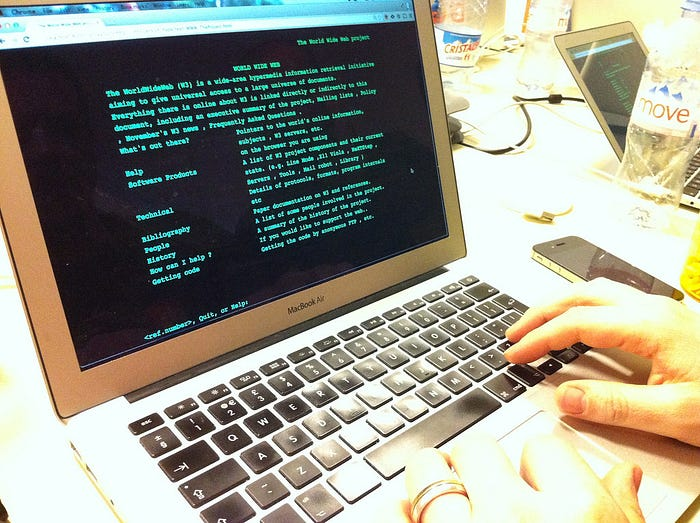In the past few posts, I have been explaining a few statistical measures, such as mean and median. There is another measure, mode, which is also pertinent to the study of statistics. The measure, mode, tells the most frequently occurring element in a list.

There are several ways to determine the mode in Python and in this post I will discuss four of those methodologies. The three ways I will cover are used in the collections library, the NumPy library, and the statistics library.

The first algorithm I will cover is by using the collections library. The pseudocode for this algorithm is as follows:

1. Import the collections library.
2. Define the function, find_mode, which takes a list of numbers as input.
3. Define the variable, data, which counts the occurrence of each element in the list.
4. Define the variable, data_list, which converts data to a dictionary.
5. Define the variable, max_value, which takes the maximum occurring value in data.
6. Define the variable, mode_val, which selects the element that has the maximum value in the data_list.
7. If the length of num_list is the same as mode_val, this indicates there is no mode, otherwise mode_val is printed out.
8. When the function is complete, it will return mode_val.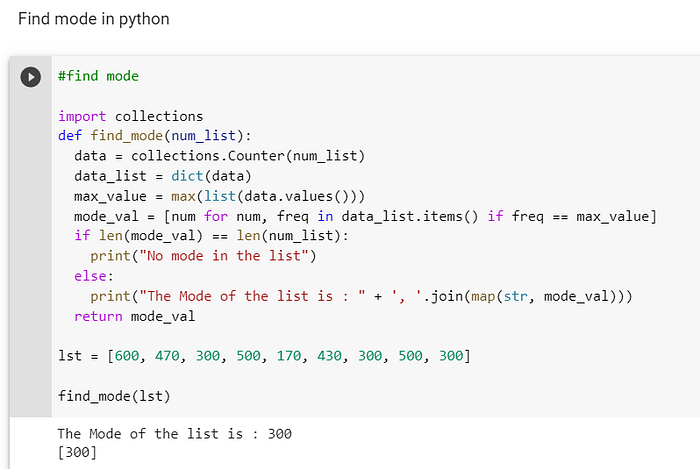The algorithm using the NumPy library is, in my opinion, slightly less complex than the method using the collections library. The pseudocode for this function is cited below:

1. Import the NumPy library.
2. Define the function, find_mode, which takes a NumPy array as input.
3. The variables vals and counts are created from the NumPy function unique, which will find the unique elements in an array and count them.
4. Define the variable index, which is derived from the NumPy method, argmax, which will elect the maximum occurring element in counts.
5. The function will then return the index of vals.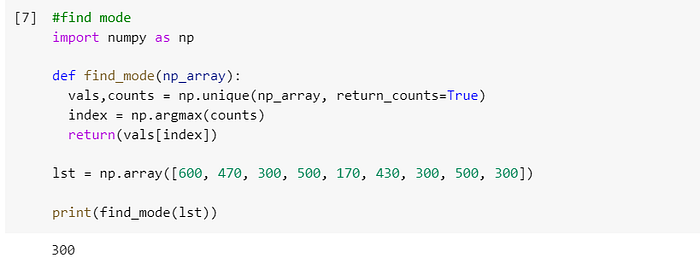The penultimate algorithm that I will discuss is the mode function that is in the in-built statistics library, which is depicted in the screenshot below: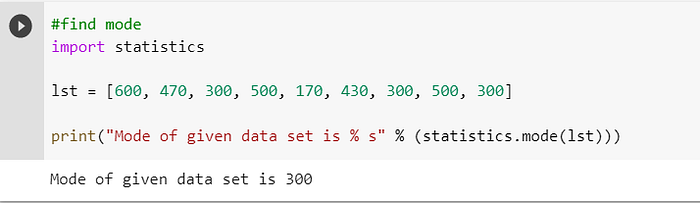The final way to find the mode is by using the pandas library, which is used to create and maintain dataframes. The mode function is part of the pandas library. The only thing that is necessary is to convert the list to a dataframe and then call up the mode function: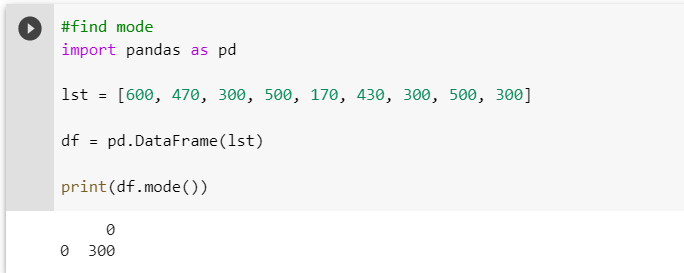I have covered four ways to find the mode in a list, NumPy array, and a dataframe. Hopefully, I equipped you, the reader, with enough information to enable you to find the mode in a list of values.

I have prepared a code review to accompany this post, which can be found here: https://www.youtube.com/watch?v=UyuYkCMHdXA